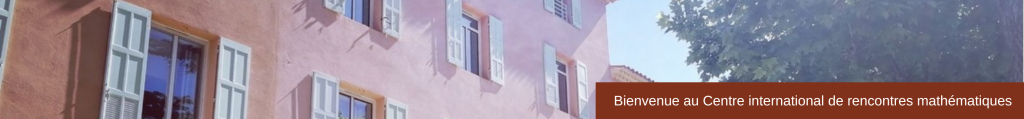Pierre Baudot (INSERM)    Information Topology: Statistical Physic of Complex Systems and Data Analysis (pdf)
Daniel Bennequin (Université Paris Diderot)   Boundary conditions in generalized belief propagation, singularities of regionalizations (pdf)
Ian Dryden (University of Nottingham)   Bayesian ambient space inference for object data (pdf)
Philippe Elbaz-Vincent (Institut Fourier) & Herbert Gangl (Durham University)   Polylogarithms, their multiple analogues and the Shannon entropy (pdf)
François Gay-Balmaz (LMD-ENS)   A Lagrangian Variational Formulation of Nonequilibrium thermodynamics (pdf)
Nathael Gozlan (Université Paris-Est)   Ehrhard’s inequality and hypercontractivity of Ornstein-Ulhenbeck semigroup (pdf)
Oliver Johnson (University of Bristol)   Entropy and discrete random variables   (pdf)
Alice Le Brigant (IMB, Université de Bordeaux)   Information geometry and shape analysis for radar signal processing (pdf)
Tom Leinster (University of Edinburgh)   The categorical origins of entropy (pdf)
Etera Livine (Laboratoire de Physique, ENS Lyon)  The Geometry of Loop Quantum Gravity (pdf)
Mokshay Madiman (University of Delaware)    Minicourse on information-theoretic geometry of metric measure spaces (pdf)
Antonino Marcianò (Fudan University)   Kac-Moody instantons in space-time foam as an alternative solution to the black hole information paradox (pdf)
Matilde Marcolli (Caltech)    The Geometry of Syntax (pdf)
Xavier Pennec (INRIA Sophia-Antipolis)  & Alain Trouvé (ENS Cachan)   Shape Spaces and Geometric Statistics (pdf)
Karol Zyczkowski (Jagiellonian University)   Geometry of Qunatum Entanglement (pdf)